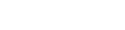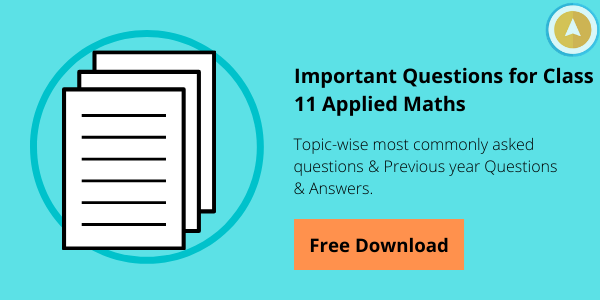• Home
• CBSE Class 11 Applie...

# CBSE Class 11 Applied Mathematics Syllabus

Author : Palak Khanna

March 3, 2022

SHARE

CBSE has introduced a new subject to their curriculum named Applied Mathematics. If you choose Commerce or Humanities stream after 10th, you can choose Applied Mathematics as optional.

To get an idea about what topics are included in the subject, this post shall take you through the detailed CBSE Class 11 Applied Maths Syllabus.

Check out the topics included and the marking scheme for all topics.

### CBSE Class 11 Applied Mathematics Marking Scheme

The below table explains the units included in class 11 applied mathematics subject. Check out the unit-wise marking scheme.

 No. Units No. of Periods Marks I. Numbers, Quantification and Numerical Applications 20 09 II. Algebra 35 10 III. Mathematical Reasoning 15 06 IV. Calculus 30 10 V. Probability 30 10 VI. Descriptive Statistics 35 12 VII Basics of Financial Mathematics 55 18 VIII Coordinate Geometry 20 05 Total 240 80 Internal Assessment - 20

### Detailed CBSE Class 11 Applied Maths Syllabus 2021

Before you begin your preparation, it is important to know the syllabus for CBSE Class 11 Applied Maths subject.

Unit I Numbers, Quantification and Numerical Applications

• Prime Numbers, Encryptions using Prime Numbers
• Binary Numbers
• Complex Numbers (Preliminary idea only)
• Indices, Logarithm and Antilogarithm
• Laws and properties of logarithms
• Simple applications of logarithm and antilogarithm
• Numerical problems on averages, calendar, clock, time, work and distance, mensuration, seating arrangementUnit II Algebra

• Sets
• Types of sets
• Venn diagram
• De Morgan's laws
• Problem-solving using Venn diagram
• Relations and types of relations
• Introduction of Sequences, Series
• Arithmetic and Geometric progression
• Relationship between AM and GM
• Basic concepts of Permutations and Combinations
• Permutations, Circular Permutations, Permutations with restrictions
• Combinations with standard results

Unit III Mathematical and Logical Reasoning

• Mathematically acceptable statements
• Connecting words/ phrases in Mathematical statement consolidating the understanding of "if and only if (necessary and sufficient) condition", "implies", "and/or", "implied by", "and", "our", "there exists" and their use through a variety of examples related to real life and Mathematics
• Problems based on logical reasoning (coding-decoding, odd man out, blood relation, syllogism, etc)

Unit IV Calculus

• Introducing functions
• Domain and Range of a function
• Types of functions (Polynomial function; Rational function; Composite function; Logarithm function; Exponential function; Modulus function; Greatest Integer function, Signum function)
• Graphical representation of functions
• Concept of limits and continuity of a function
• Instantaneous rates of change
• Differentiation is a process of finding derivative
• Derivatives of algebraic functions using the Chain rule
• Tangent line and equations of tangents

Unit V Probability

• Random experiment, sample space, events, mutually exclusive events
• Independent and Dependent Events
• Law of Total Probability
• Bayes’ Theorem

Unit VI Descriptive Statistics

• Types of data (raw data, univariate data, bivariate and multivariate data)
• Data on various scales (nominal, ordinal, interval, and ratio scale)
• Data representation and visualization
• Data interpretation (central tendency, dispersion, deviation, variance, skewness, and kurtosis)
• Percentile rank and quartile rank
• Correlation (Pearson and Spearman method of correlation)
• Applications of descriptive statistics using real-time data

Unit VII Basics of Financial Mathematics

• Interest and interest rate
• Accumulation with simple and compound interest
• Simple and compound interest rates with equivalency
• The effective rate of interest
• Present value, net present value, and future value
• Annuities, calculating the value of the regular annuity
• Simple applications of regular annuities (up to 3 periods)
• Tax, calculation of tax and simple applications of tax calculation in Goods and service tax, Income Tax
• Bills, tariff rates, fixed charge, surcharge, service charge
• Calculation and interpretation of electricity bill, water supply bill, and other supply bills

(Comparing interest rates on various types of savings; calculating income tax; electricity bills, water bill; service surcharge using realistic data)

Unit VIII Coordinate Geometry

• Straight Line
• Circles
• Parabola

(only standard forms and graphical representation on two-dimensional plane)

Practical: Use of spreadsheet

Calculating average, interest (simple and compound), creating pictographs, drawing pie chart, bar graphs, calculating central tendency; visualizing graphs (straight line, circles, and parabola using real-time data)

Suggested practical using spreadsheet

1. Plot the graph of functions on excel; study the nature of function at various points, drawing lines of tangents;
2. Create a budget of income and spending;
3. Create compare sheet of price, features to buy a product;
4. Prepare the best option plan to buy a product by comparing cost, shipping charges, tax, and another hidden cost;
5. Smart purchasing during sale season;
6. Prepare a report card using scores of the last four exams and compare the performance;
7. Collect the data on weather, price, inflation, and pollution. Sketch different types of graphs.

Download CBSE 11th Applied Maths Syllabus PDF

Numerical analysis, mathematics of engineering, linear programming, optimization and operations research, continuous modelling, mathematical biology and bioinformatics, information theory, game theory, probability and statistics, financial mathematics, actuarial science, cryptography are some of the branches of Applied Mathematics.
The subjects included in Class 12 Applied Mathematics are:

• Numbers, Quantification and Numerical Applications,
• Algebra,
• Mathematical Reasoning,
• Calculus,
• Probability
• Descriptive Statistics,
• Basics of Financial Mathematics, and
• Coordinate Geometry.

Pure mathematics is used to solve the problems related to mathematics and applied mathematics is used to answer the questions related to various fields like physics, biology, economics, and so on.
Yes, there are many applied maths courses online available which provide in-depth information about each subject. Also, offer quality coaching for students from which they can score more marks.
• Home
• CBSE Class 11 Applie...

# CBSE Class 11 Applied Mathematics Syllabus

Author : Palak Khanna

Updated On : March 3, 2022

SHARE

CBSE has introduced a new subject to their curriculum named Applied Mathematics. If you choose Commerce or Humanities stream after 10th, you can choose Applied Mathematics as optional.

To get an idea about what topics are included in the subject, this post shall take you through the detailed CBSE Class 11 Applied Maths Syllabus.

Check out the topics included and the marking scheme for all topics.

### CBSE Class 11 Applied Mathematics Marking Scheme

The below table explains the units included in class 11 applied mathematics subject. Check out the unit-wise marking scheme.

 No. Units No. of Periods Marks I. Numbers, Quantification and Numerical Applications 20 09 II. Algebra 35 10 III. Mathematical Reasoning 15 06 IV. Calculus 30 10 V. Probability 30 10 VI. Descriptive Statistics 35 12 VII Basics of Financial Mathematics 55 18 VIII Coordinate Geometry 20 05 Total 240 80 Internal Assessment - 20

### Detailed CBSE Class 11 Applied Maths Syllabus 2021

Before you begin your preparation, it is important to know the syllabus for CBSE Class 11 Applied Maths subject.

Unit I Numbers, Quantification and Numerical Applications

• Prime Numbers, Encryptions using Prime Numbers
• Binary Numbers
• Complex Numbers (Preliminary idea only)
• Indices, Logarithm and Antilogarithm
• Laws and properties of logarithms
• Simple applications of logarithm and antilogarithm
• Numerical problems on averages, calendar, clock, time, work and distance, mensuration, seating arrangementUnit II Algebra

• Sets
• Types of sets
• Venn diagram
• De Morgan's laws
• Problem-solving using Venn diagram
• Relations and types of relations
• Introduction of Sequences, Series
• Arithmetic and Geometric progression
• Relationship between AM and GM
• Basic concepts of Permutations and Combinations
• Permutations, Circular Permutations, Permutations with restrictions
• Combinations with standard results

Unit III Mathematical and Logical Reasoning

• Mathematically acceptable statements
• Connecting words/ phrases in Mathematical statement consolidating the understanding of "if and only if (necessary and sufficient) condition", "implies", "and/or", "implied by", "and", "our", "there exists" and their use through a variety of examples related to real life and Mathematics
• Problems based on logical reasoning (coding-decoding, odd man out, blood relation, syllogism, etc)

Unit IV Calculus

• Introducing functions
• Domain and Range of a function
• Types of functions (Polynomial function; Rational function; Composite function; Logarithm function; Exponential function; Modulus function; Greatest Integer function, Signum function)
• Graphical representation of functions
• Concept of limits and continuity of a function
• Instantaneous rates of change
• Differentiation is a process of finding derivative
• Derivatives of algebraic functions using the Chain rule
• Tangent line and equations of tangents

Unit V Probability

• Random experiment, sample space, events, mutually exclusive events
• Independent and Dependent Events
• Law of Total Probability
• Bayes’ Theorem

Unit VI Descriptive Statistics

• Types of data (raw data, univariate data, bivariate and multivariate data)
• Data on various scales (nominal, ordinal, interval, and ratio scale)
• Data representation and visualization
• Data interpretation (central tendency, dispersion, deviation, variance, skewness, and kurtosis)
• Percentile rank and quartile rank
• Correlation (Pearson and Spearman method of correlation)
• Applications of descriptive statistics using real-time data

Unit VII Basics of Financial Mathematics

• Interest and interest rate
• Accumulation with simple and compound interest
• Simple and compound interest rates with equivalency
• The effective rate of interest
• Present value, net present value, and future value
• Annuities, calculating the value of the regular annuity
• Simple applications of regular annuities (up to 3 periods)
• Tax, calculation of tax and simple applications of tax calculation in Goods and service tax, Income Tax
• Bills, tariff rates, fixed charge, surcharge, service charge
• Calculation and interpretation of electricity bill, water supply bill, and other supply bills

(Comparing interest rates on various types of savings; calculating income tax; electricity bills, water bill; service surcharge using realistic data)

Unit VIII Coordinate Geometry

• Straight Line
• Circles
• Parabola

(only standard forms and graphical representation on two-dimensional plane)

Practical: Use of spreadsheet

Calculating average, interest (simple and compound), creating pictographs, drawing pie chart, bar graphs, calculating central tendency; visualizing graphs (straight line, circles, and parabola using real-time data)

Suggested practical using spreadsheet

1. Plot the graph of functions on excel; study the nature of function at various points, drawing lines of tangents;
2. Create a budget of income and spending;
3. Create compare sheet of price, features to buy a product;
4. Prepare the best option plan to buy a product by comparing cost, shipping charges, tax, and another hidden cost;
5. Smart purchasing during sale season;
6. Prepare a report card using scores of the last four exams and compare the performance;
7. Collect the data on weather, price, inflation, and pollution. Sketch different types of graphs.

Download CBSE 11th Applied Maths Syllabus PDF

Numerical analysis, mathematics of engineering, linear programming, optimization and operations research, continuous modelling, mathematical biology and bioinformatics, information theory, game theory, probability and statistics, financial mathematics, actuarial science, cryptography are some of the branches of Applied Mathematics.
The subjects included in Class 12 Applied Mathematics are:

• Numbers, Quantification and Numerical Applications,
• Algebra,
• Mathematical Reasoning,
• Calculus,
• Probability
• Descriptive Statistics,
• Basics of Financial Mathematics, and
• Coordinate Geometry.

Pure mathematics is used to solve the problems related to mathematics and applied mathematics is used to answer the questions related to various fields like physics, biology, economics, and so on.
Yes, there are many applied maths courses online available which provide in-depth information about each subject. Also, offer quality coaching for students from which they can score more marks.